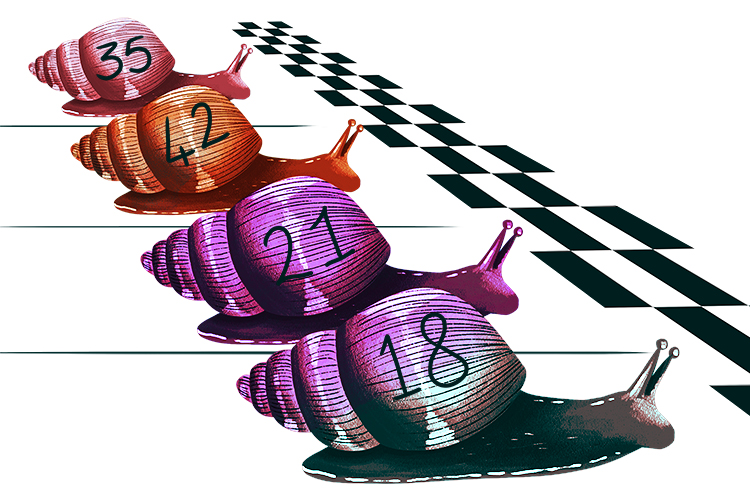# Snell's law

Some exam boards require you to understand Snell's law.

To do this first you have to know that:

REFRACTIO has  an  iNdex  called N
N = Refractive index

This refraction law relates to our definition of refraction.

REFerence ACTION (Refraction)and the reference action is a SNAIL RACE.SNAILS RACE = SNELL'S LAW

And as well as all the above.

SNAILS RACE
has SIN in it

Which reminds you that there is a sin rule too. This sin rule is:

(sin\ \i\n\ \theta)/(sin\ \out\ \theta)=(n\ \(out))/(n\ \(i\n))

NOTE: It will be easy to remember that n\ \(out) is on the top on the other side when later you find that n\ \(i\n) is the value of 1 most of the time.

## Summary

Refraction reminds you:

RefractioN  and  iNdex  = N = Refractive index

Refraction reminds you

REFerence ACTION = SNAILS RACE = SNELL'S LAW

SNAILS RACE reminds you

(sin\ \i\n\ \theta)/(sin\ \out\ \theta)=(n\ \(out))/(n\ \(i\n))